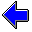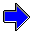Java Security Settings:
This web page employs Java, which requires specific security settings for correct operation.
If the applets on this page do not run correctly, consult the Virtual Chemistry Experiments FAQ
or the Physlet Physics web site for establishing the correct security settings.

# Chemical Kinetics

## Rate of Reaction

### Concepts

In the previous exercise the concentration-time plots showed that each species in the reaction has its own rate of change in concentration. The reactants have a negative slope, because they are being consumed in the reaction. Products have a positive slope, because they are being formed in the reaction. For the hypothetical example reaction

A + 2 B   →   3 C

the stoichiometric coefficient for species B is twice that of species A; thus the concentration of B will decline twice as fast as that of species A. Similarly, the concentration of species C increases three times as fast as the concentration of A decreases.

Conceptually there should be a single, unambiguous rate for a reaction. How might such a rate be defined given the highly varied rates of change for the various species in the reaction?

The rate of reaction, r, is defined to be the slope of the concentration-time plot for a species divided by the stoichiometric coefficient of that species. Additionally, if the species is a reactant, the negative value of the slope is used, because the slope is negative and a positive rate is desired. For the example shown above

 rate of reaction = r = - d [A]d t = - 12 d [B]d t = 13 d [C]d t

Notice that this definition produces the same rate of reaction irrespective of which species in the reaction is used to perform the calculation.

### Part 1. Determination of the Rate of Reaction

Objective

• Determine the rate of reaction at a given point in time.

This stopped-flow experiment involves the following reaction:

2 A + 3 B   →   5 C

Run the simulation and use the controls to select various points on the concentration-time plot. Use the numerical values for the slope at each point to determine the rate of reaction at 0, 4, and 7 seconds. (Note that the rate of reaction changes as time progresses.)

To plot the slope and value of the slope, click on one of the options A, B, C or 0 sec, 4 sec, 7 sec.

 Species A B C Time 0 sec 4 sec 7 sec slope (mole L-1 sec-1)

### Part 2. Determination of the Stoichiometric Coefficients

Objective

• Determine the stoichiometric coefficients for a chemical reaction.

As is evident from the above example, there is a relationship between the stoichiometric coefficient of a species in a reaction, the rate of change of the concentration of that species, and the rate of reaction. This relationship can be exploited to determine the stoichiometric coefficients for a chemical reaction.

In this experiment, the chemical reaction is described by the following chemical equation.

a A + b B   →   c C

The lower case letters are stoichiometric coefficients. Your goal in this experiment is to determine the values of a, b, and c by comparing the various slopes of the concentration-time plots for species A, B, and C. The comparison is based upon the requirement that all slopes must ultimately produce the same rate of reaction.

 r = - 1a d [A]d t = - 1b d [B]d t = 1c d [C]d t

 Species A B C Time 0 sec 4 sec 7 sec slope (mole L-1 sec-1)Reaction Rates                     Differential Rate LawsChemical Kinetics Home PageVirtual Chemistry Home Page

RateOfReaction.html version 2.1
© 2000-2014 David N. Blauch